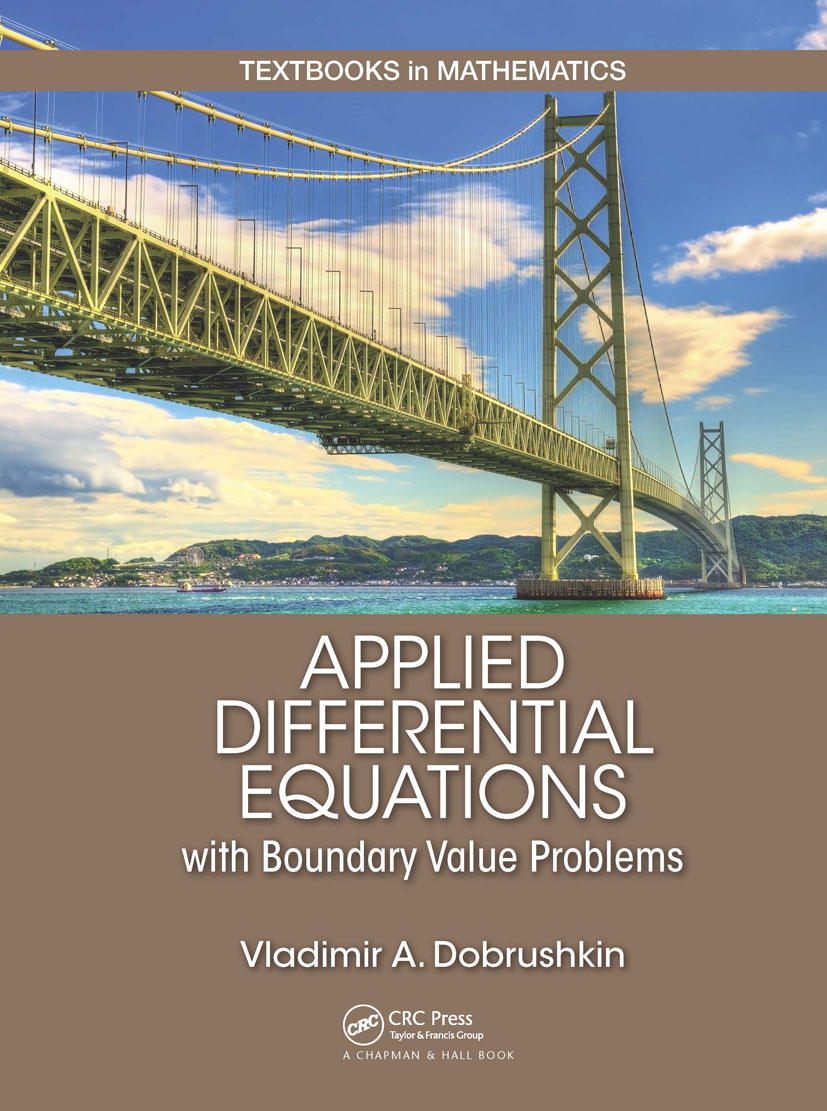# Applied Differential Equations with Boundary Value Problems

## 1st Edition

Chapman and Hall/CRC

684 pages | 260 B/W Illus.

##### Purchasing Options:\$ = USD
Hardback: 9781498733656
pub: 2017-10-16
SAVE ~\$25.00
\$125.00
\$100.00
x
eBook (VitalSource) : 9781315369785
pub: 2017-10-19
from \$62.50

FREE Standard Shipping!

### Description

Applied Differential Equations with Boundary Value Problems presents a contemporary treatment of ordinary differential equations (ODEs) and an introduction to partial differential equations (PDEs), including their applications in engineering and the sciences. This new edition of the author’s popular textbook adds coverage of boundary value problems.

The text covers traditional material, along with novel approaches to mathematical modeling that harness the capabilities of numerical algorithms and popular computer software packages. It contains practical techniques for solving the equations as well as corresponding codes for numerical solvers. Many examples and exercises help students master effective solution techniques, including reliable numerical approximations.

This book describes differential equations in the context of applications and presents the main techniques needed for modeling and systems analysis. It teaches students how to formulate a mathematical model, solve differential equations analytically and numerically, analyze them qualitatively, and interpret the results.

### Table of Contents

First-Order Equations. Applications of First Order ODE. Mathematical Modeling and Numerical Methods. Second-order Equations. Laplace Transforms. Series of Solutions. Applications of Higher Order Differential Equations. Linear Boundary Value Problems, Introduction to Green's Functions; Nonhomogeneous Boundary Value Problems; Singular Sturm--Liouville Problems, Bessel Series Expansion, Orthogonal Polynomial Expansions; Appendix: Software Packages. Answers to Problems. Bibliography. Index

### About the Author

Vladimir A. Dobrushkin is a Visiting Professor in the Center for Fluid Mechanics—Applied Mathematics Department at Brown University.

### Subject Categories

##### BISAC Subject Codes/Headings:
MAT000000
MATHEMATICS / General
MAT003000
MATHEMATICS / Applied
MAT007000
MATHEMATICS / Differential Equations